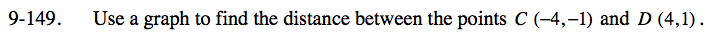Home > CC3 > Chapter 9 > Lesson 9.2.7 > Problem9-149

9-149.

Use a graph to find the distance between the points C(−4, −1) and D(4, 1). 9-149 HW eTool (Desmos). Homework Help ✎Turn line CD into a right triangle, with legs parallel to the x- and y-axes.

Use the Pythagorean Theorem to find the length of the line segment connecting the two points.

82 + 22 = c2
64 + 4 = c2
68 = c2

${\it c} = \sqrt{68} \approx 8.25$

Complete the table in the eTool below to graph the points.
Click the link at right for the full version of the eTool: CC3 9-149 HW eTool